### IMO Shortlist 2002 problem G7

Kvaliteta:
Avg: 0,0
Težina:
Avg: 9,0
The incircle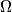$\Omega$ of the acute-angled triangle$ABC$ is tangent to its side$BC$ at a point$K$. Let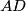$AD$ be an altitude of triangle$ABC$, and let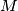$M$ be the midpoint of the segment$AD$. If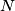$N$ is the common point of the circle$\Omega$ and the line$KM$ (distinct from$K$), then prove that the incircle$\Omega$ and the circumcircle of triangle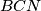$BCN$ are tangent to each other at the point$N$.
Izvor: Međunarodna matematička olimpijada, shortlist 2002